Laying out multiple plots on a page

2019-07-13

An individual ggplot object contains multiple pieces – axes, plot panel(s), titles, legends –, and their layout is defined and enforced via the gtable package, itself built around the lower-level grid package. Plots themselves become graphical objects, which can be arranged on a page using e.g. the gridExtra or egg packages, which provide helper functions for such multi-object layouts. The following schematic illustrates the main relations between these packages.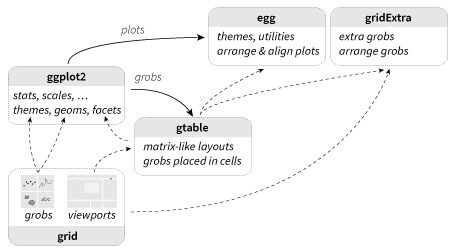Schematic illustration of the links between packages ggplot2, gtable, grid, egg and gridExtra.

Arranging multiple plots on a page

To begin, we’ll create four example plots that we can experiment with.

library(ggplot2)
p1 <- qplot(mpg, wt, data = mtcars, colour = cyl)
p2 <- qplot(mpg, data = mtcars) + ggtitle("title")
p3 <- qplot(mpg, data = mtcars, geom = "dotplot")
p4 <-
p1 + facet_wrap( ~ carb, nrow = 1) + theme(legend.position = "none") +
ggtitle("facetted plot")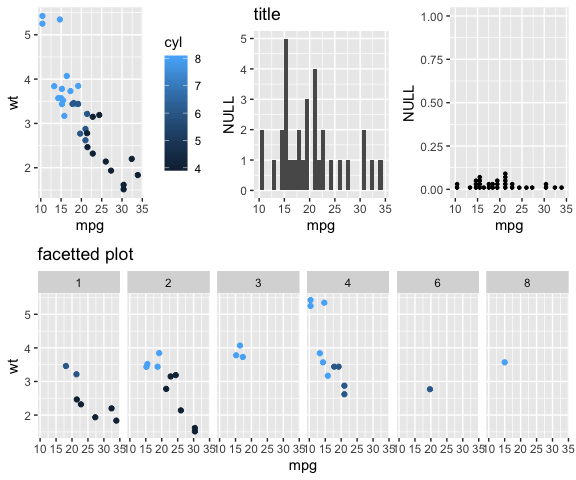A few plots that we want to organise on a page.

Basic strategy

The easiest approach to assemble multiple plots on a page is to use the grid.arrange() function from the gridExtra package; in fact, that’s what we used for the previous figure. With grid.arrange(), one can reproduce the behaviour of the base functions par(mfrow=c(r,c)), specifying either the number of rows or columns,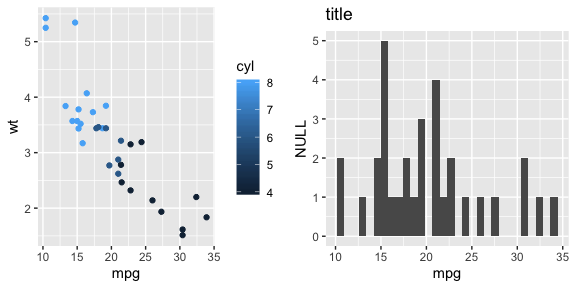Basic usage of grid.arrange()

If layout parameters are ommitted altogether, grid.arrange() will calculate a default number of rows and columns to organise the plots.

More complex layouts can be achieved by passing specific dimensions (widths or heights), or a layout matrix defining the position of each plot in a rectangular grid. For the sake of clarity, we’ll use a list gl of dummy rectangles, but the process is identical for plots.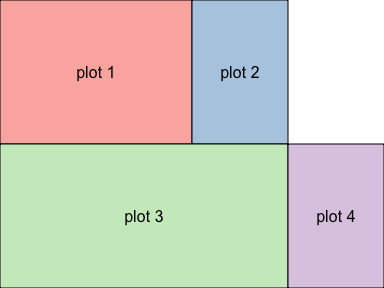Illustrating further arguments of grid.arrange(), namely layout_matrix and relative widths.

Further examples are available in a dedicated gridExtra vignette.

Plot insets

A special case of layouts is where one of the plots is to be placed within another, typically as an inset of the plot panel. In this case, grid.arrange() cannot help, as it only provides rectangular layouts with non-overlapping cells. Instead, a simple solution is to convert the plot into a grob, and place it using annotation_custom() within the plot panel. Note the related geom_custom() function, suitable when different facets should display different annotations.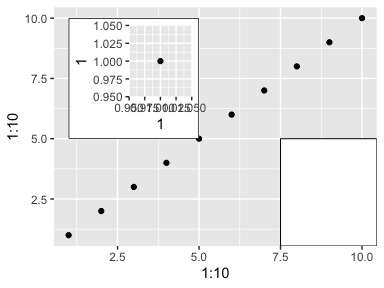Plot inset.

In the second annotation, we used the convenient shorthand +/-Inf to signify the edge of the plot, irrespective of the data range.

An alternative way to place custom annotations within the plots is to use raw grid commands, which we will present at the end of this document. However, note that an advantage of using annotation_custom is that the inset plot is embedded in the main plot, therefore the whole layout can be saved with ggsave(), which will not be the case for plot modifications at the grid level.

Aligning plot panels

A common request for presenting multiple plots on a single page is to align the plot panels. Often, facetting the plot solves this issue, with a flexible syntax, and in the true spirit of the Grammar of Graphics that inspired the ggplot2 design. However, in some situations, the various plot panels cannot easily be combined in a unique plot; for instance when using different geoms, or different colour scales.

grid.arrange() makes no attempt at aligning the plot panels; instead, it merely places the objects into a rectangular grid, where they fit each cell according to the varying size of plot elements. The following figure illustrates the typical structure of ggplots.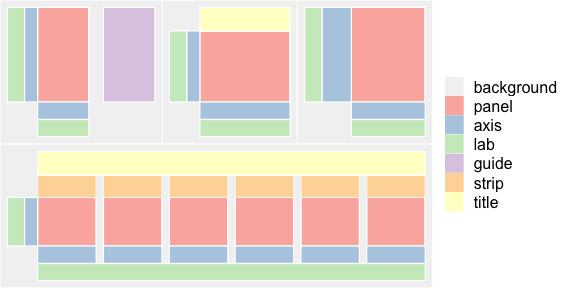Colour-coded structure of examplar ggplot layouts. Note how the panels (red) vary in size from plot to plot, as they accommodate the other graphical components.

As we can readily appreciate, each plot panel stretches or shrinks according to the other plot elements, e.g. guides, axes, titles, etc. This often results in misaligned plot panels.

In this situation, instead of using grid.arrange(), we recommend to switch to the more powerful gtable package. In particular, the rbind(), cbind(), and join functions can provide a better alignment. The plots must first be converted to grobs (more specifically, gtables), using the ggplotGrob() function. The second step is to bind the two gtables, using the sizes from the first object, then assigning them to the maximum. Finally, the resulting object, a gtable, can be displayed using grid.draw() (it is no longer a ggplot, so print() no longer renders it on a device).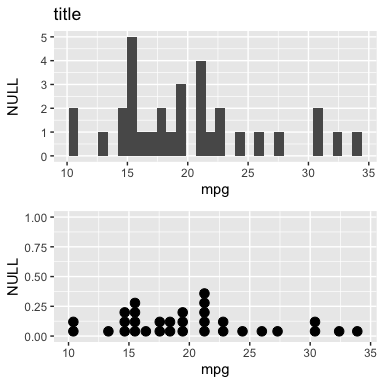Aligning plot panels. Note that the two y axes have different widths.

Aligning complex plots

One possible strategy, implemented in egg with the low-level gtable_frame and high-level ggarrange functions, is to take the following steps:

• decompose each plot into a 3x3 layout, where the central cell corresponds to the core panels, surrounded by axes, legends, etc.
• set the core width and height to a fixed dimension
• bind the individual 3x3 gtables

Aligning plots is achieved simply as follows,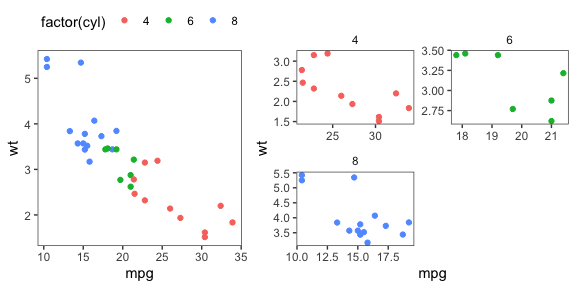where many parameters are common to the grid.arrange() function from gridExtra.

Multiple pages?

Plots produced by ggplot2, including those with facets, and those combined with grid.arrange(), are always displayed on a single page. Sometimes, however, there isn’t enough room to display all the information, and it becomes necessary to split the output on multiple pages. A convenient approach consists in storing all the plots in a list, and plotting subsets of them on subsequent pages. The gridExtra package can simplify this process with the helper function marrangeGrob(), sharing a common syntax with grid.arrange(), but outputting as many pages as required by the total number of plots and per-page layout.

Mixed graphical components

Titles and subtitles

Adding a global title and/or subtitle to a page with multiple plots is easy with grid.arrange(): use the top, bottom, left, or right parameters to pass either a text string, or a grob for finer control.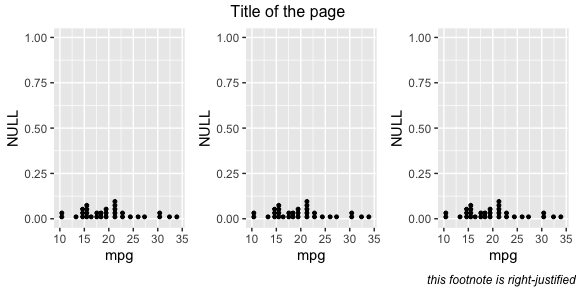Recent versions of ggplot2 have added built-in options to add a subtitle and a caption; the two stategies are somewhat complementary (grid.arrange aligns elements with respect to the entire plot, whereas ggplot2 places them with respect to the plot panel area).

Legends

When arranging multiple plots, one may wish to share a legend between some of them (although in general this is a clear hint that facetting might be a better option). The procedure involves extracting the legend from one graph, creating a custom layout, and inserting the plots and legend in their corresponding cell.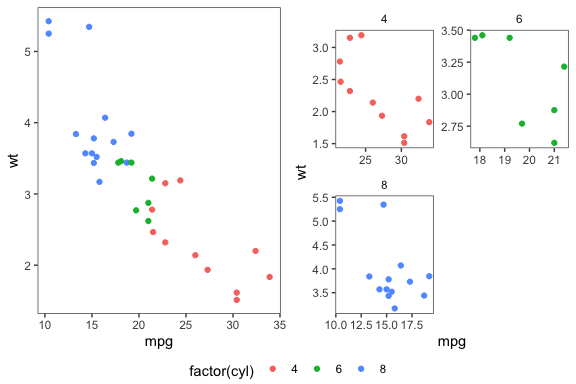Tables and other grobs

As we’ve seen in the previous examples, ggplots are grobs, which can be placed and manipulated. Likewise, other grobs can be added to the mix. For instance, one may wish to add a small table next to the plot, as produced by the tableGrob function in gridExtra.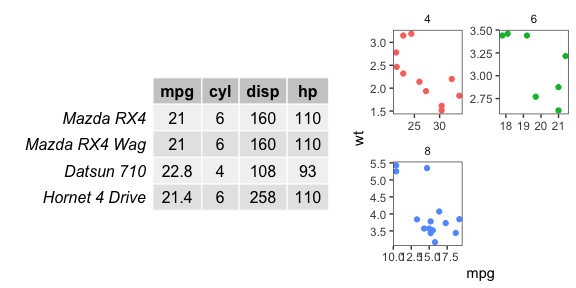Alternative options

We’ve focused on grid.arrange() and ggarrange for simplicity, but there are numerous alternatives to achieve similar arrangements of plots (all of which ultimately based on grid). We list below a few alternatives, in chronological order.

Package Function(s) ggsave compat. alignment
grid viewport, grid.layout no no
gridExtra grid.arrange yes no
(r cookbook) multiplot no no
gtable rbind, cbind yes yes
cowplot plot_grid yes* yes*
multipanelfigure multi_panel_figure yes yes
egg ggarrange yes yes
patchwork plot_layout yes yes

grid viewports and layouts

Underlying all these other packages is the grid package, included in the core R distribution. This package provides the low-level functions used for drawing and placing objects on a device.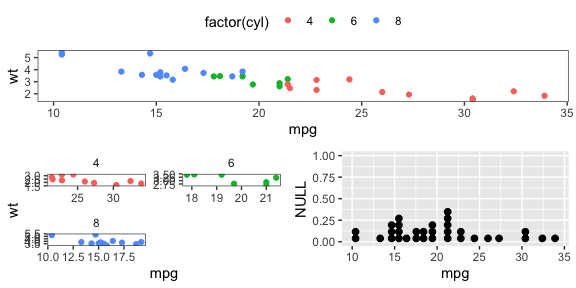Acknowledgements

Parts of this document were originally written by Hadley Wickham as a draft vignette for the ggplot2 package; the contents have since been shuffled around quite a bit and I have lost track of the exact history of edits.